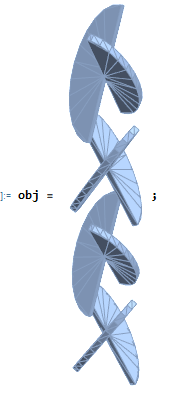# Volume measurements on 3D objects

When creating objects for 3D printing, I find I often want to know the volume. In one particular instance, I am making what is called a low pressure drop static mixer and would like to know what volume of a channel is with the mixer installed. Turns out that the problem is easy to solve, so long as you know the right tricks.

First, here’s a closer look at the type of mixer I want to create. It’s a series of semi-elliptical plates that are positioned perpendicular to one another. Each pair of plates are supposed to split and then divert the stream. If the input stream consists of two miscible liquids undergoing laminar flow, then the mixer will split the two streams into $L=2\times2^n$ streams where n is the number of elements (so 64 streams for the object below).A LPD static mixer insert. The four bars are not part of the mixer, but are present to support the mixing elements.

I constructed a 4-element channel using OpenSCAD (the code is at the end of this post in case you are interested.). The plates (which I call fins in the code) aren’t perpendicular but at an angle of 60 degrees. I was just messing around with the code and noted that this angle resulted in a successful 3D print so I haven’t yet returned to the “correct” angle. Here’s the draft design:40 mm channel with 4 LPD mixing elements (60 degree). Channel diameter is 4.6 mm and nominal plate diameter is 5 mm.

I wanted to know what the channel volume was with the mixers inserted. Mathematica can measure the volumes of objects very easily, but it turns out there is a problem in measuring the volume of an STL object because it is technically a set of polygons (2-dimensional objects) in 3D space. Therefore, Mathematica doesn’t know what to do with this object.

I found the solution over at Stack Overflow and was able to convert the accepted answer into Mathematica code very easily:

Clear[stlVolume];
stlVolume[mesh_MeshRegion] := Module[{mp},
mp = MeshPrimitives[mesh, 2];
#[].Cross[#[], #[]]/6.0 & /@ mp[[All, 1]] // Total
]

Here, I am taking the imported STL file (which Mathematica treats as a MeshRegion and extract all of the polygon primitives. Then we apply $\frac{p_1\cdotp (p_2 \times p_3)}{6.0}$ to each primitive and totals those values. [Note: eventually, I found an answer on the Mathematica Stack Exchange site which is very similar to what I proposed.]LDP elements generated in OpenSCAD and imported into Mathematica

Four elements have a volume of 77 $\mu L$; however, I intentionally added some overlap between the elements and the inner channel so the elements would fuse to the channel. Therefore, to get a better estimate of the channel volume, I found the volume of the solid object and subtracted the volume of the actual object that was to be printed. The result was about 592 $\mu L$ vs 658 $\mu L$ for an empty channel. Nice!

Now, I need to see if a 3D printed LPD static mixer will actually mix. I know that the channel can print, but I need to create an object that has a few entry/exit ports before I can actually do the test. Stay tuned.

OpenSCAD code for creating the LPD static mixer

module fin(a = 45,flip = false, ccw = false, diameter=10, thickness=1) {
rotate((ccw?-1:1)*a,[0,1,0])mirror([0,flip?1:0,0])scale([diameter/sin(90-a),diameter,thickness])difference(){
cylinder(d=1,h=1,$fn=25,center=true); translate([0,0.5,0])cube([1,1,1.1],center=true); } } module lbdunit(a = 60, diameter=5, thickness=0.5, separation=4){ translate([0,0,separation])rotate(90,[0,0,1]){ fin(flip=true,ccw=true,diameter=diameter,thickness=thickness,a=a); fin(ccw=false,diameter=diameter,thickness=thickness,a=rot); } translate([0,0,-separation]){ fin(flip=true,ccw=false,diameter=diameter,thickness=thickness,a=a); fin(ccw=true,diameter=diameter,thickness=thickness,a=a); } } for(i=[8,-8]){ translate([0,0,i])lbdunit(); } color("red",0.4) difference(){ cylinder(d=10,h=40,center=true,$fn=6);
cylinder(d=4.6,h=41,center=true);
}

This site uses Akismet to reduce spam. Learn how your comment data is processed.